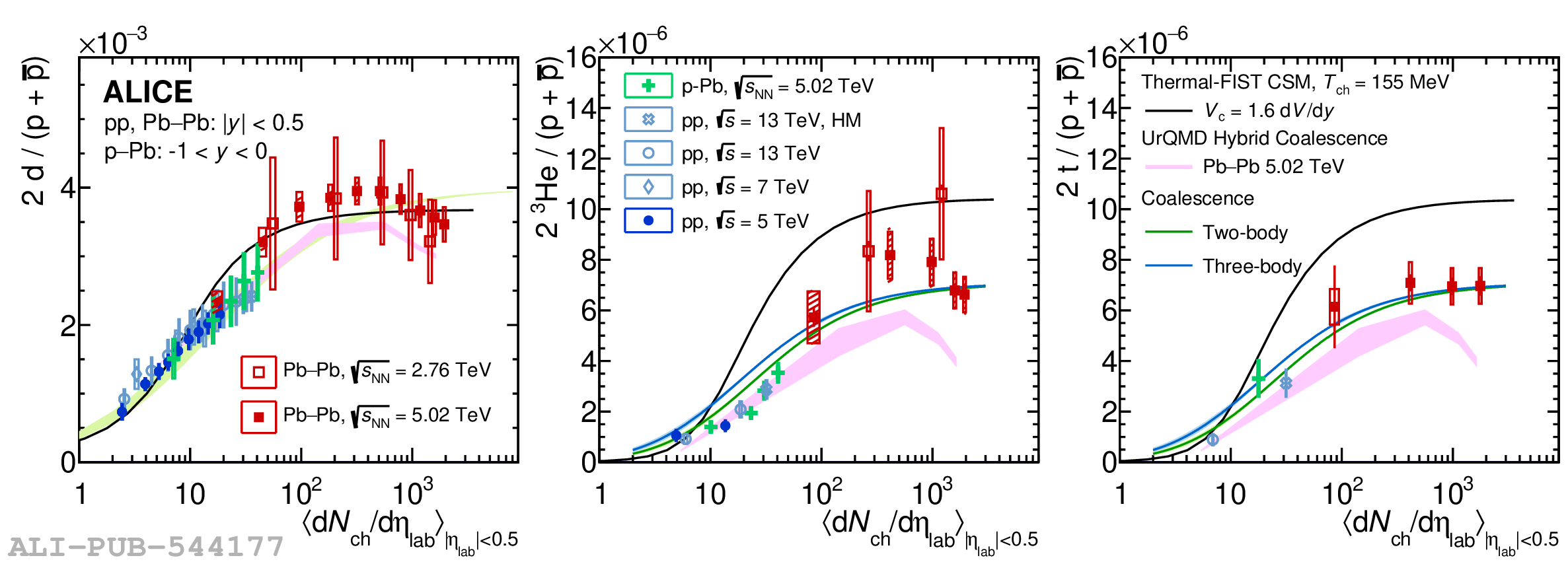# Figure 7

 Integrated deuteron (left), \Hee (middle) and triton yields (right) over proton yields as a function of charged-particle multiplicity $\langle\textrm{d}N/\textrm{d}\eta_{\textrm{ch}}\rangle$ for pp, p--Pb and Pb--Pb collisions measured by the ALICE Collaboration. The boxes represent the uncorrelated systematic uncertainties, while the vertical lines are the statistical ones. The shaded boxes represent the centrality-correlated uncertainties. In addition, the data is compared to the Thermal-FIST CSM (canonical statistical model) at 155\,MeV with a correlation volume of $V_\mathrm{c} = 1.6 {\rm d}V/{\rm d}y$ shown as black line , the two coalescence approaches displayed in green (two-body coalescence) and in blue (three-body coalescence)  and UrQMD hybrid coalescence shown as purple line .